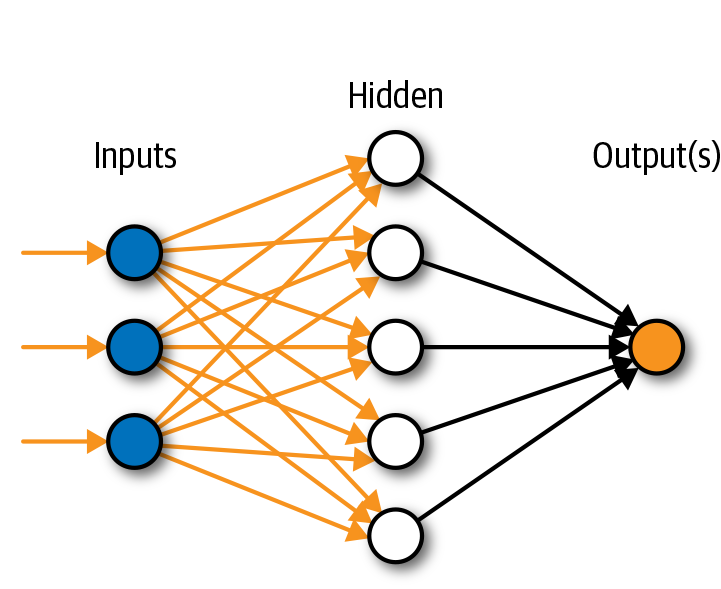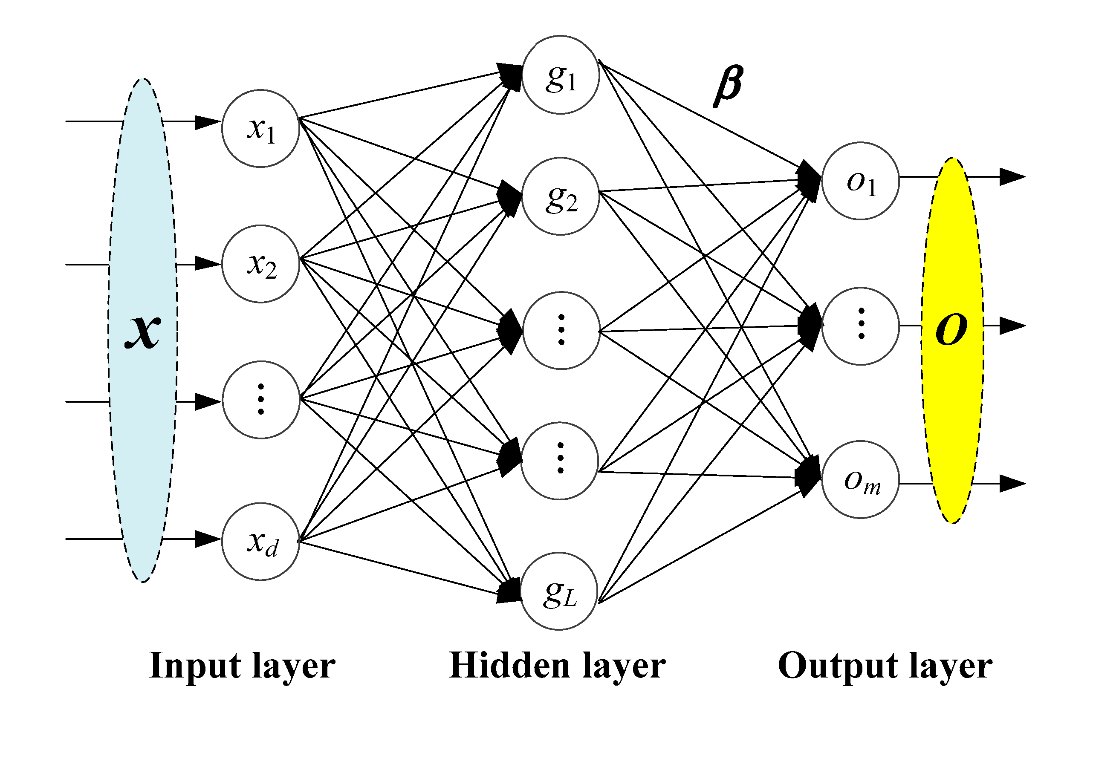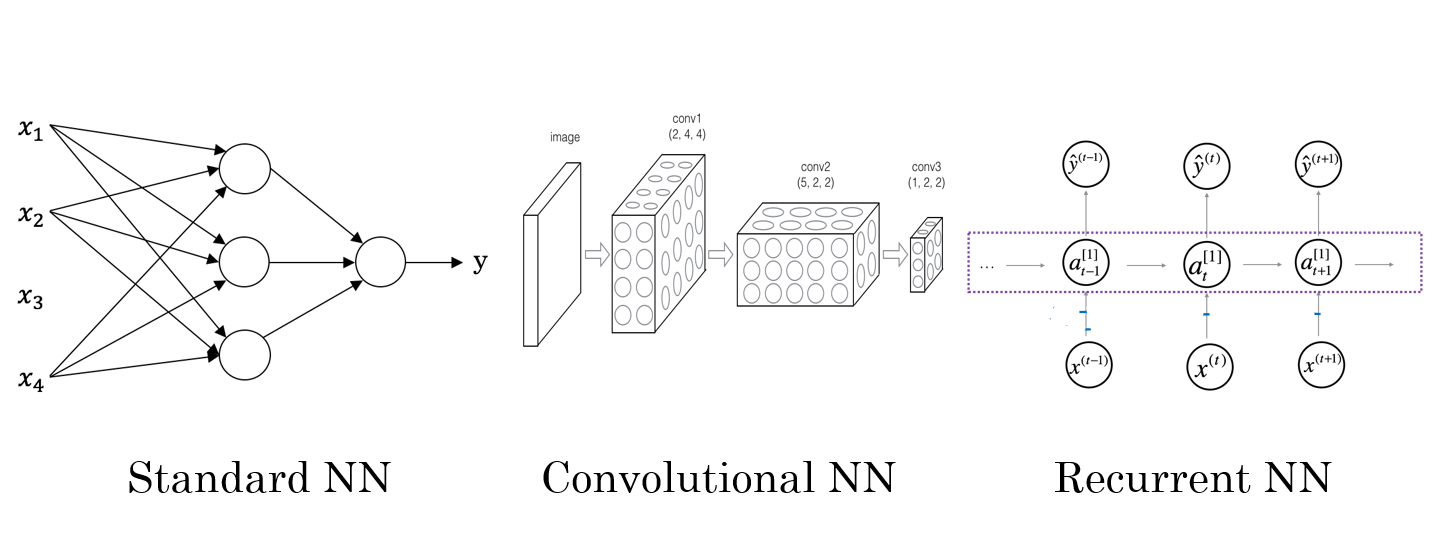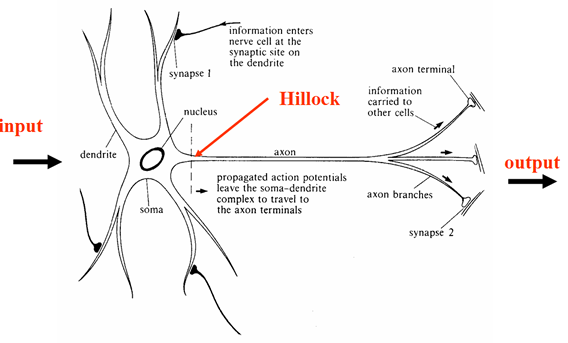##### What is an artificial neural network (ANN)?

Artificial neural networks (ANNs) are a subset of machine learning at the core of deep learning algorithms.

An artificial neuron network (neural …

Feb. 2, 2022 7 minuteArtificial neural networks (ANNs) are a subset of machine learning at the core of deep learning algorithms.

An artificial neuron network (neural network) is a computational model that mimics how nerve cells work in the human brain to give machines the ability to recognize patterns, process data similarly to the brain, and make decisions.

ANNs help machines complete and learn from their tasks even though they have yet to reach the same imagination and reasoning power as humans. Deep learning ANNs complement machine learning (ML) and artificial intelligence (AI).

• Why is Artificial Neural Network critical?
• What are the components of an Artificial Neural Network?
• How does Artificial Neural Network work?
• Types of Artificial Neural Network
• Future of Artificial Neural Network

## What is the importance of an artificial neural network?

A neural network can also help people solve complex problems in real-life situations. Nonlinear and complex inputs and outputs can be learned and modeled; generalizations and inferences can be made; hidden relationships, patterns, and predictions can be revealed; and highly volatile data (such as financial time series data) and variances can be calculated to predict rare events (such as fraud detection).

Consequently, neural networks can improve decision-making processes in areas such as:

• Fraud detection for credit cards and Medicare
• Diagnose medical conditions and diseases
• Control systems for robots
• Natural language processing, also known as character recognition and voice recognition
• In computer vision, raw photos and videos are interpreted (for example, in medical imaging, robotics, and facial recognition)
• Forecasting of electrical load and energy demand.

## What are the components of an Artificial Neural Network?

A simple neural network has three main layers: input, processing, and output. Weighting may be applied to inputs based on a variety of factors. In the processing layer, hidden from view, nodes and connections between them are analogous to neurons and synapses in an animal brain.## How does an artificial neural network work?

A neural network combines several processing layers, using simple elements that operate in parallel. The network has three layers: an input layer, a hidden layer, and an output layer.

In each layer, there are several nodes or neurons, and the nodes in each layer use the outputs of the nodes in the previous layer as inputs, allowing the neurons to communicate through the layers. In the learning process, neuronal weights are typically adjusted; decreases or increases in the weight affect the signal strength of that neuron.

As with other machine learning algorithms, neural networks can be used for supervised learning (classification, regression) and unsupervised learning (pattern recognition, clustering).

Machine learning determines model parameters by optimizing weights to minimize prediction errors by weighting the neural network on training data.## Types of artificial neural networks

There are different types of deep neural networks, each with advantages and disadvantages. Examples include:

Frank Rosenblatt created the Perceptron neural network in 1958, the oldest neural network. This is the simplest form of the neural network, with only one neuron.

A convolutional neural network (CNN) consists of five layers: input, convolution, pooling, fully connected, and output. Each layer serves a specific purpose, such as summarizing, connecting, or activating.

Convolutional neural networks have popularized image classification and object detection. CNNs have also been applied to natural language processing and forecasting, among other areas.

Sequential information, such as time-stamped data from a sensor device or words in a spoken sentence, is used by recurrent neural networks (RNNs).

Recurrent neural networks differ from traditional neural networks in that their inputs are not independent, and their output depends on the computations of their predecessors. Fore­casting and time series applications, sentiment analysis, and other text applications use RNNs.

The feedforward neural network connects every perceptron at one layer to every perceptron at the next layer. Layers feedforward information only in the forward direction. In this system, there are no feedback loops.

From a set of inputs, autoencoder neural networks create abstractions called encoders. As opposed to traditional neural networks, autoencoders attempt to model the inputs themselves, so they are unsupervised methods—Autoencoders work by desensitizing the irrelevant and sensitizing the relevant.

The abstractions are formulated at higher layers (layers closest to the point at which a decoder layer is introduced). Classifiers can then use these abstractions, whether they are linear or nonlinear.

While ANNs can handle most tasks if trained, their main challenges are their lengthy training process and the amount of computation required for a complex task. Furthermore, humans cannot fully comprehend what happens in an artificial neural network's hidden layers. Although researchers are actively working on this, there is still a lot to learn about making machines think and act like humans.Future of artificial neural network

Which programming language is for artificial intelligence and neural network?

The Smalltalk programming language has been extensively used for simulations, neural networks, and machine learning. It implements the highest and purest form of object-oriented programming through message passing.

R is widely used in artificial intelligence, including statistical computations, numerical analysis, Bayesian inference, neural networks, and general machine learning. Finance, biology, sociology, and medicine are the main standard languages. It offers several programming paradigms like vectorial computation and functional and object-oriented programming.

The Python programming language is widely used for artificial intelligence, with various packages for applications such as General Artificial Intelligence, Machine Learning, Natural Language Processing, and Neural Networks. Machine Learning uses AI to develop programs that mimic human skills and perform tasks like humans. Machine Learning and Artificial Intelligence are closely related and widely used today.

How to build an artificial neural network?See both images above. The first image shows a typical biological neuron. Information enters the neuron through the input or dendrite, and these inputs can also be seen in the second image with the values (x1,..., XM). A weight (w1,...,wm) is assigned to each input in the artificial neural network model.

We use these weights to determine how essential inputs are for training; the higher the weight, the more critical the input. As a result, all inputs are added together (Σ) and enter the axon as a single layer. Next, we apply the Activation Function to the data. The activation Function is defined according to the needs of the problem and the type of our neural network.

A mathematical formula is used to update the weights in the network in this function. After performing the calculations in this step, our information enters another neuron through output synapses, and this step continues until the network is trained.## Conclusion

Today's world is the world of learning machines. Hundreds of machines now recognize your personality from your searches, correct your spelling and writing errors by guessing what you mean and learn and grow like humans in different situations.

Scientists could no longer achieve this level of learning with typical processors and programming. For this reason, they designed a system similar to the human brain, which can learn and grow just like a baby. The artificial neural network is currently used in a variety of industries.